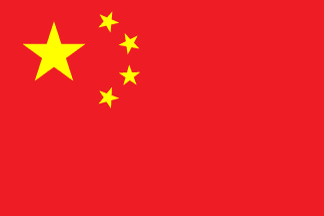###China

 A Hu, Q. Q Xu, Q.Y. An, M. J Qin, T.R. Xu, T. Ao, L Jia, D. R Xu, X. B Jin, Y. Renjie, L. Y Bai, X. K S Yan, H. Bai, Y.M. Kong, F. Shao, S.Q. Yan, X.P. Bao, J. L Shen, C. Yang, C. Bi, X.Y. Lee, J.H. Shi, C.J. Yao, X. C Li, G. Sun, Y. Yong, J. Cai, F. Li, L.N. T Yu, C.Y. Cai, Y. Li, M.F. Tu, X. Yu, J. Chang, L. Li, T.W. W Yu, L. Chen, G. Li, W. Wang, D.L. Yu, Q. Chen, G. Li, Z. Wang, D. Z Chen, H. Liao, B.J. Wang, F.W. Zhan, F. Chen, J. Liu, B. Wang, N. Zhang, C.F. Chen, J. Liu, C.G. Wang, S.Z. Zhang, D. Chen, P.F. Liu, H. Wang, T.F. Zhang, J.F. Chen, T. Liu, K. Wang, X.H. Zhang, J. Chen, X. Liu, R. Wang, Y. Zhang, J.F. Chen, X. Liu, T. Wang, Z. Zhang, M.Y. Chen, Y. Liu, W. Wang, Z.W. Zhang, M.M. Cheng, W. Liu, X. Wei, S. Zhang, R.L. Chu, X.M. Liu , X.J. Wei, Y. Zhang, W. D Liu, Y.Q. Wei, Z. Zhang, X. Ding, J. Liu, Z.J. Wen, Y.Q. Zhang, Y. Dong, W.H. Lv, J. Wu, B. Zhao, C. F M Wu, H. Zhao, C.C. Feng, H. Ma, F. Wu, J. Zhao, D. Fu, J. Ma, H. Wu, Q. Zhao , J.S. G Ma, J. Wu, S. Zhao, J.S. Geng, H. Ma, N. Wu, Z.L. Zhao, J.J. Geng, J. Man, Y. X Zheng, Z. Geng, X. Meng, X.Y. Xiang, Z. Zhou , C.H. Gu, X.C. Mo, J.Y. Xiao, B. Zhou, G. Guan, K. Mou, J.M. Xiao, C.S. Zhou, P. H N Xiao, Y. Zhou, X.Y. Han, X.J. Nie, Y. Xie, Z.X. Zhou, X. He, H. P Xin, X. Zhu, M. He , Y.K. Peng, J. Xu, M. Zhu, Z.